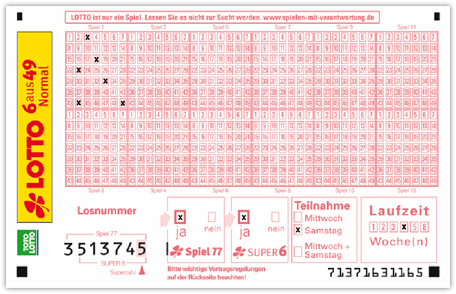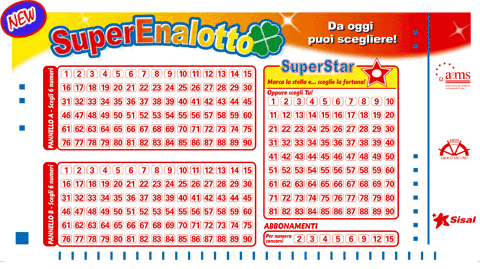#### Loops and calculations

Figure 174. Calculating values
 final int LIMIT = 5; int sum = 0; for (int i = 1; i <= LIMIT; i++) { sum += i; } System.out.println("1 + ... + " + LIMIT + " = " + sum); 1 + ... + 5 = 15No. 81

##### Display all summands

Q:

In Figure 174, “Calculating values ” for a given value of limit only the first and last summand will show up: We just see 1 + ... + 5 = 15 rather than 1 + 2 + 3 + 4 + 5 = 15.

If LIMIT is equal to one the visible result is even worse:

1 + ... + 1 = 1

Modify the code accordingly to print the above 1 + 2 + 3 + 4 + 5 = 15 output line.

### Tip

Using System.out.print(...) rather than System.out.println(...) avoids printing a newline and thus allows for continuation within a given line of output.

A:

final int LIMIT = 5;

for (int i = 1; i <= LIMIT; i++) {
System.out.print(i);
System.out.print(" + ");
sum += i;
}

System.out.println(" = " + sum);

This is close to the intended output. However our sum ends with an excess trailing + symbol:

1 + 2 + 3 + 4 + 5 +  = 15

We get rid of it by introducing an if statement:

final int LIMIT = 5;

for (int i = 1; i <= LIMIT; i++) {
System.out.print(i);
if (i < LIMIT) {            // Avoid '+' for the very last value
System.out.print(" + ");
}
sum += i;
}

System.out.println(" = " + sum);

This avoids the trailing +:

1 + 2 + 3 + 4 + 5 = 15

Moreover it works for LIMIT = 1 as well:

1 = 1

Instead of filtering the very last + operator we may as well terminate our loop one step earlier and move the last operand to the second println(...) statement leaving us with an identical result:

final int LIMIT = 5;

int sum = 0;
for (int i = 1; i < LIMIT; i++) {
System.out.print(i + " + ");
sum += i;
}

System.out.println(LIMIT + " = " + (sum + LIMIT));No. 82

##### Playing lottery

Q:

Common lotteries randomly draw numbers from a given set like:

Germany / Glücksspirale
 6 out of 49Italy / SuperEnalotto
 6 out of 90This category of lottery does not care about ordering (apart from so called jolly numbers). The number of possibilities for drawing k out of n numbers ignoring their ordering is being given by the binomial coefficient $( n k )$:

$( n k ) = n ! k ! ⁢ ( n - k ) !$

Write an application which allows for determining the probabilistic success rates using this coefficient. For the German Glücksspirale a possible output reads:

Your chance to win when drawing 6 out of 49 is 1 : 13983816

Store parameters like 6 or 49 in variables to keep your software flexible.

### Tip

1. Why is it a bad idea to e.g. compute $49!$ directly even when using long variables? Remember Figure 104, “Arithmetic overflow pitfalls ”.

2. Arithmetic overflow problems can be avoided by simplifying $n ! k ! ⁢ ( n - k ) !$ beforehand. You may want to write down an explicit example like $( 8 3 ) = 8 ! 3 ! ⁢ ( 8 - 3 ) !$ to learn about cancellation of contributing factors.

A:

Consider the following snippet:

int product = 1;
for (int i = 1; i < 50; i++) {
product *= i;
System.out.println(i + "! == " + product);
}

This results in:

1! == 1
2! == 2
3! == 6
4! == 24
5! == 120
6! == 720
7! == 5040
8! == 40320
9! == 362880
10! == 3628800
11! == 39916800
12! == 479001600
13! == 1932053504
...
49! == 0

Only results up to $12!$ are correct: The next term $13!$ equals 6227020800 which is ways beyond Integer.MAX_VALUE == 2147483647 giving rise to a (silent) arithmetic overflow. But even declaring our variable product of type long does not help much:

1! == 1
2! == 2
3! == 6
4! == 24
5! == 120
6! == 720
7! == 5040
8! == 40320
9! == 362880
10! == 3628800
11! == 39916800
12! == 479001600
13! == 6227020800
14! == 87178291200
15! == 1307674368000
16! == 20922789888000
17! == 355687428096000
18! == 6402373705728000
19! == 121645100408832000
20! == 2432902008176640000
21! == -4249290049419214848
...
49! == 8789267254022766592

This time 20! == 2432902008176640000 is the last correct value being smaller than Long.MAX_VALUE == 9223372036854775807.

Fortunately we have another option. Consider an example:

$( 8 3 ) = 8 × 7 × 6 × 5 × 4 × 3 × 2 × 1 ( 3 × 2 × 1 ) × ( 5 × 4 × 3 × 2 × 1 ) = 8 × 7 × 6 3 × 2 × 1 = 56$

We generalize this fraction cancellation example:

Equation 1. Calculating binomials by cancelling common factors.
$n ! k ! ⁢ ( n - k ) ! = n ⁢ ( n - 1 ) ⁢ ... ⁢ 1 k ⁢ ( k - 1 ) ⁢ ... ⁢ 1 ⁢ ( n - k ) ⁢ ( n - k - 1 ) ⁢ ... ⁢ 1 = n ⁢ ( n - 1 ) ⁢ ... ⁢ ( n - k + 1 ) k ⁢ ( k - 1 ) ⁢ ... ⁢ 1$

And thus:

$( 49 6 ) = 49 × 48 × 47 × 46 × 45 × 44 6 × 5 × 4 × 3 × 2 × 1 = 13983816$

We calculate numerator and denominator in two separate loops:

public class Lottery {

public static void main(String[] args) {

// Input values:
final int
totalNumberCount = 49,
drawnNumberCount = 6;

// No changes below this line

// Numerator loop
long numerator = 1;
for (int i = totalNumberCount - drawnNumberCount + 1;
i <= totalNumberCount; i++) {
numerator *= i;
}

// Denominator loop calculating the "smaller" factorial
long factorial = 1;
for (int i = 2; i <= drawnNumberCount; i++) {
factorial *= i;
}

System.out.println("Your chance to win when drawing " + drawnNumberCount +
" out of " + totalNumberCount + " is 1 : " + (numerator / factorial));
}
}